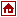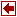Author: IBM corp.

Description

MPS was the first step towards the advanced modeling languages. From the user viewpoint MPS is like an assembling languages in comparison with the programming languages. This leads to great difficulties to write manually large-scale linear programming models. Mainly, the translator associated to the current modeling language have a special function to generate the equivalent MPS form of the model. Usualy the commercial solvers admit as input the MPS form of the model. Besides this, in the integrated systems of modeling and optimization, there is another internal form of communication between the associated translator of the modeling language and the optimizers, which is not transparent to the user.

MPS INPUT FORMAT (excerpted from Advanced Linear Programming: Computation and Practice by Bruce A. Murtagh, McGraw-Hill, INC., 1981.)

This format has become the industry standard, adopted by most commercial codes, and is not restricted to the MPS series of codes developed originally for IBM computers.

Table: MPS format data line

Field 1 Field 2 Field 3 Field 4 Field 5 Field 6
Columns 2 - 3 5 - 12 15 - 22 25 - 36 40 - 47 50 - 61
Contents Indicator Name Name Value Name Value

The various sections of the data are grouped in the following order:

• NAME
• ROWS
• COLUMNS
• RHS
• RANGES (optional)
• BOUNDS (optional)
• ENDATA

We will examine each of these sections in turn.

1.NAME. This section consists of just one card, with the word NAME in columns 1-4, and the title of the problem in columns 15-22.

2.ROWS. In this section all the row labels are defined, as well as the row type. The row type is entered in field 1 (in column 2 or 3) and the row label is entered in field 2 (columns 5-12).

The code for specifying row type is as follows:

Row type Indicator col. 2 or 3 Description
= E equality
<= L less than or equal
>= G greater than or equal
Objective N objective
Free N no restriction

This section of data is preceded by a card with ROWS in columns 1-4, followed by a data card for each row. The first N-type row encountered is regarded as the objective, unless it is explicitly identified in the control commands.

Linear combinations of rows may also be specified. In this case the above row types are denoted respectively by the codes DE, DL, DG, and DN, in columns 2-3. Field 2 contains the linear combination rowname. Fields 3-6 contain the rowname(s) (fields 3 and 5) and their multiplier(s) (fields 4 and 6) which form the combination. A linear combination of three or more rows requires additional cards, following the first card contiguously. In the additional cards field 1 is empty. (The right-hand sides of a linear combination row must be specified in the RHS section, described below.)

3.COLUMNS. This section defines the names of the variable, the coefficients of the objective, and all the nonzero matrix elements aij. The data are entered column by column, and all the data cards for the nonzero entries in each column must be grouped together contiguously. The section is preceded by a card with COLUMNS in columns 1-7, followed by data cards which may have one or two matrix elements per card.

The data card has the column label in field 2 (columns 5-12), the row label in field 3 (columns 15-22), and the value of the coefficient aij(or cj) in field 4 (columns 25-36), including a decimal point). If more than one nonzero row entry for the same column is to be made on the card, then field 5 (columns 40-47) has the next row label and field 6 (columns 50-61) has its corresponding coefficient value. It should be emphasized that the use of fields 5 and 6 is optional.

There is no need to specify columns for slack variables; this is taken care of automatically having defined the row types.

4.RHS. This section contains the elements of the right-hand side. The section is preceded by a card with RHS in columns 1-3. Since the right- hand side can be regarded as another column of the matrix, the data cards specifying the nonzero entries are in exactly the same format as the COLUMNS data cards, except field 2 (columns 5-12) has a label for the right-hand side. More than one right-hand side may thus be specified in this section; the one to be used for the current run is specified by its label in the agenda cards, to be discussed in Sec 9.3.

5.RANGES (optional). This section is for constraints of the following form:

hi <= ai1 x1 + ai2 x2 + ... ain xn <= ui i.e., both an upper and lower bound exist for the row. The range of the constraint is ri = ui - hi .The value of or is specified in the RHS section data, and the value of ri is specified in the RANGES section data. This information, plus the row type specified in the ROWS section, defines the bounds ui and hi .

If bi is the number entered in the RHS section and is the number specified in the RANGES section, the ui and hi are defined as follows.

Row type Sign of ri Lower limit hi Upper limit ui
G(>=) + or - bi bi + |ri|
L(<=) + or - bi - |ri| bi
E(=) + bi bi + |ri|
E(=) - bi - |ri| bi

The section is preceded by a card with RANGES in columns 1-6. The data cards specifying the values of ri are in exactly the same format as the COLUMNS data cards, except field 2 (columns 5-12) has a label for the column of ranges (which can also be regarded as another column of the matrix). More than one column of ranges may be specified, but all the data cards for each column must be grouped together contiguously.

6.BOUNDS (optional). In this section, bounds on the variables are specified. The section is preceded by a card with BOUNDS in columns 1-6. The bounds are entered as a row, with a corresponding row label. The nonzero entries in this row vector correspond to columns in the matrix and must be in the same order in which the column names appear in the COLUMNS section. When bounds are not specified for a column ( or the entire BOUNDS section omitted) the usual bounds,

0 <= xj < ¥, are assumed.

More than one bound for a particular variable may be entered, i.e., both a lower and an upper bound; when only one is specified the other is assumed to be one of the default values of 0 or ¥ as shown in parentheses below.

Field 1 (columns 2-3) specifies the type of bound:

Type Description Mathematic
LO Lower bound 0 <= xj (< ¥)
UP Upper bound (0 <=) xj <= bj
FX Fixed variable xj = bj
FR Free variable - ¥ < xj < + ¥
MI Loer bound - - ¥ < xj <= 0
PL Upper bound + (default bound) (0 <= xj ) < ¥

Field 2 (columns 5-12) specifies the bounds row label. (More than one bounds row may be entered, but the data must be grouped together contiguously for each bounds row.)

Field 3 (columns 15-22) specifies the column label (j)corresponding to the variable xj

Field 4 (columns 25-36) specifies the bound value bj

Fields 5 and 6 are blank.

7.ENDATA.This section is just one card, with ENDATA in columns 1-6, signalling the end of matrix data input.

Example

```NAME AFIRO
ROWS
E  R09
E  R10
L  X05
L  X21
E  R12
E  R13
L  X17
L  X18
L  X19
L  X20
E  R19
E  R20
L  X27
L  X44
E  R22
E  R23
L  X40
L  X41
L  X42
L  X43
L  X45
L  X46
L  X47
L  X48
L  X49
L  X50
L  X51
N  COST
COLUMNS
X01 X48   .301 R09  -1.
X01 R10  -1.06 X05  1.
X02 X21  -1.   R09  1.
X02 COST -.4
X03 X46  -1.   R09  1.
X04 X50  1.    R10  1.
X06 X49  .301  R12  -1.
X06 R13  -1.06 X17  1.
X07 X49  .313  R12  -1.
X07 R13  -1.06 X18  1.
X08 X49  .313  R12  -1.
X08 R13  -.96  X19  1.
X09 X49  .326  R12  -1.
X09 R13  -.86  X20  1.
X10 X45  2.364 X17  -1.
X11 X45  2.386 X18  -1.
X12 X45  2.408 X19  -1.
X13 X45  2.429 X20  -1.
X14 X21  1.4   R12  1.
X14 COST -.32
X15 X47  -1.   R12  1.
X16 X51  1.    R13  1.
X22 X46  .109  R19  -1.
X22 R20  -.43  X27  1.
X23 X44  -1.   R19  1.
X23 COST -.6
X24 X48  -1.   R19  1.
X25 X45  -1.   R19  1.
X26 X50  1.    R20  1.
X28 X47  .109  R22  -.43
X28 R23  1.    X40  1.
X29 X47  .108  R22  -.43
X29 R23  1.    X41  1.
X30 X47  .108  R22  -.39
X30 R23  1.    X42  1.
X31 X47  .107  R22  -.37
X31 R23  1.    X43  1.
X32 X45  2.191 X40  -1.
X33 X45  2.219 X41  -1.
X34 X45  2.249 X42  -1.
X35 X45  2.279 X43  -1.
X36 X44  1.4   R23  -1.
X36 COST -.48
X37 X49  -1.   R23  1.
X38 X51  1.    R22  1.
X39 R23  1.    COST 10.
RHS
B   X50  310.  X51  300.
B   X05  80.   X17  80.
B   X27  500.  R23  44.
B   X40  500.
BOUNDS
ENDATA
```

References

• Fourer, R., Modeling languages versus matrix generators for linear programming. ACM-TOMS, vol.9, No.2, June 1983, pp.143-183.
• Fourer, R., Database structures for mathematical programming models. Technical Report 90-06, Northwestern University , Evanston, Illinois 60208-3119.
• Murphy, F.H., Sthor, E.S., Asthana, A., Representation schemes for linear programming models. Management Science, vol.38, No.7, July, 1992, pp.964-991.